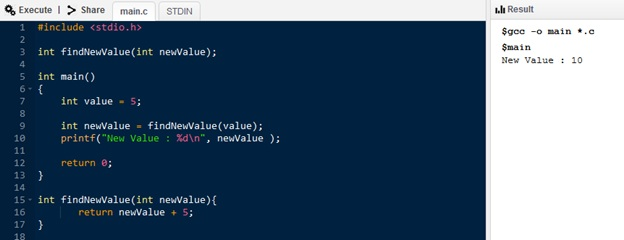# What is the Difference Between Pass by Value and Pass by Reference

The main difference between pass by value and pass by reference is that, in a pass by value, the parameter value copies to another variable while, in a pass by reference, the actual parameter passes to the function.

A computer program is a set of instructions that directs the CPU to perform a certain task. There are various concepts in programming to write efficient and effective programs. One of them is function, which is a group of reusable statements. Rather than writing all the statements in the same program, it is possible to divide it into several functions and call them in the main program. That makes the programs more manageable and easy to maintain. In this, there are two methods of calling a function. They are by using pass by value or pass by reference.

### Key Areas Covered

1. What is Pass by Value
– Definition, Functionality
2. What is Pass by Reference
– Definition, Functionality
3. What is the Difference Between Pass by Value and Pass by Reference
– Comparison of Key Differences

### Key Terms

Pass by Value, Pass by Reference## What is Pass by Value

In pass by value, the value of a function parameter is copied to another location of the memory. When accessing or modifying the variable within the function, it accesses only the copy. Thus, there is no effect on the original value.Figure 1: C program with pass by value

In the program illustrated above, the variable value stores integer 5. The findNewValue is a function. The value is passed to that function. In the function, the value is then copied to a new memory location called newValue. The function then returns an integer. And, this integer is stored in the newValue variable of the main function. Finally, the newValue is printed on the console.

In the function, the value copied to a new memory location is called newValue. The changes are made to that newValue, not to the original value. This method is called Pass by Value.

## What is Pass by Reference

In pass by reference, the memory address is passed to that function. In other words, the function gets access to the actual variable. An example is as follows.Figure 2: C program with pass by reference

The variable value stores integer 5. The findNewValue is a function. The address of the memory location ‘value’ is passed to that function. Thus, the function gets this value. The newValue is a pointer. It points to the original memory location called value. The function adds 5 to the original value pointed by newValue. Then, the calculated value is returned and stored into the newValue variable. Finally, the newValue is printed on the console.

In this method, the memory location passes to the function. Therefore, the changes are made to the original value. Hence, this method is called Pass by Reference.

## Difference Between Pass by Value and Pass by Reference

### Definition

Pass by value refers to a mechanism of copying the function parameter value to another variable while the pass by reference refers to a mechanism of passing the actual parameters to the function. Thus, this is the main difference between pass by value and pass by reference.

### Changes

In pass by value, the changes made inside the function are not reflected in the original value. On the other hand, in pass by reference, the changes made inside the function are reflected in the original value. Hence, this is another difference between pass by value and pass by reference.

### Actual Parameter

Moreover, pass by value makes a copy of the actual parameter. However, in pass by reference, the address of the actual parameter passes to the function.

### Association with Function

Another difference between pass by value and pass by reference is that, in pass by value, the function gets a copy of the actual content whereas, in pass by reference, the function accesses the original variable’s content.

### Memory Requirement

Besides, the pass by value requires more memory than pass by reference.

### Time Requirement

Time requirement is one other difference between pass by value and pass by reference. Pass by value requires more time as it involves copying values whereas pass by reference requires a less amount of time as there is no copying.

### Conclusion

The main difference between pass by value and pass by reference is that, in pass by value, the parameter value copies to another variable while in pass by reference, the actual parameter passes to the function.

##### Reference:

1. “Functions in C – Javatpoint.” Www.javatpoint.com, Available here.